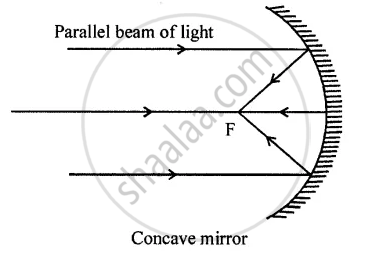# How is a spherical mirror used to converge a beam of light at a point? Name the type of mirror used. - Physics

Diagram

How is a spherical mirror used to converge a beam of light at a point? Name the type of mirror used.

#### Solution

If a parallel beam of light is incident on a concave mirror, it converges the beam to a point called focusType of mirror used is CONCAVE.

Concept: Spherical Mirrors
Is there an error in this question or solution?

#### APPEARS IN

Selina Concise Physics Class 8 ICSE
Chapter 5 Light Energy
Short/long Answer Question | Q 29 | Page 100